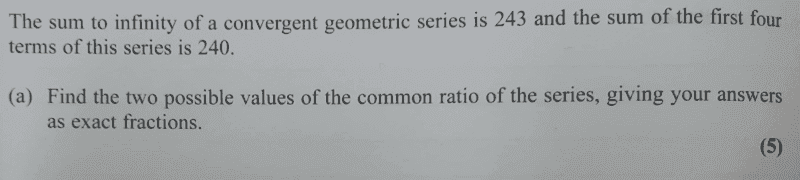# Find the two values of the common ratio- Geometric sequence

Gold Member
Homework Statement:
See attached.
Relevant Equations:
sequences*kindly note that i do not have the solutions ...

I was looking at this, not quite sure on what they mean by exact fractions, anyway my approach is as follows;

##\dfrac {a}{243}=\dfrac{a(1-r^3)}{240}##

##\dfrac {1}{243}=\dfrac{1-r^3}{240}##

##\dfrac {240}{243}=1-r^3##

##r^3=1-\dfrac{240}{243}##

##r^3=\dfrac{3}{243}##

##r=\dfrac{1}{\sqrt 81}=\dfrac{1}{3\sqrt 3}##

My alternative approach,
##(243-243r)(1-r^3)=240-240r##
##243r^4-243r^3-3r+3=0## using ti- nspire i realized same value as first approach...

I am not sure of my answer, I will need to check the r value and see whether i will realize the sum of ##240##. On quick checking i seem to have the correct terms i.e ##[184.4..., 43.6333..., 9.85468..., 2.2813...]## whose ##S_{4}≅240.##

I think i got it...let me post my working...From
##r=\dfrac{1}{\sqrt 81}=\dfrac{1}{3\sqrt 3}##,

We shall have;

##r_{1}=\dfrac{1}{3}## and ##r_{2}=\dfrac{1}{\sqrt 3}##

On checking with, ##r=\dfrac{1}{3}## , we have the terms of the sequence as ##[162,54,18,6 ]## which add up to ##240##. Cheers guys.

Last edited:

Gold Member
$$a_0+a_1+a_2+a_3+... =a_0\frac{1}{1-r}=\frac{1}{243}$$
$$a_0+a_1+a_2+a_3=a_0\frac{1-r^4}{1-r}=\frac{1}{240}$$
give ##r^2=1/9##.

Last edited:
•chwala
Gold Member
$$a_0+a_1+a_2+a_3+... =a_0\frac{1}{1-r}=\frac{1}{243}$$
$$a_0+a_1+a_2+a_3=a_0\frac{1-r^4}{1-r}=\frac{1}{240}$$
give ##r^2=1/9##.
Does that mean my approach is wrong? Is my ##r_2## correct? Of course, we only have one ##r## value that will satisfy our problem. Thanks @anuttarasammyak

Last edited:
Mentor
not quite sure on what they mean by exact fractions
Exact fraction: 1/3
Not exact fraction: .333
IOW, not numbers like 9.85468...
chwala said:
##\dfrac {a}{243}=\dfrac{a(1-r^3)}{240}##
I believe you're off here. The numerator on the right side should be ##a(1 - r^4)##. If you fix that error, you get what @anuttarasammyak got, ##r^2 = \frac 1 9##, which has two solutions.
Does that mean my approach is wrong?
Yes, see above.

•chwala
Gold Member
Exact fraction: 1/3
Not exact fraction: .333
IOW, not numbers like 9.85468...

I believe you're off here. The numerator on the right side should be ##a(1 - r^4)##. If you fix that error, you get what @anuttarasammyak got, ##r^2 = \frac 1 9##, which has two solutions.

Yes, see above.
silly me...was fixated on using nth term formula ...##r^{n-1}## instead of sum... ##r^n##...i did this in a rush...cheers Mark.

Gold Member
Homework Statement:: See attached.
Relevant Equations:: sequences

View attachment 301873

*kindly note that i do not have the solutions ...

I was looking at this, not quite sure on what they mean by exact fractions, anyway my approach is as follows;

##\dfrac {a}{243}=\dfrac{a(1-r^3)}{240}##

##\dfrac {1}{243}=\dfrac{1-r^3}{240}##

##\dfrac {240}{243}=1-r^3##

##r^3=1-\dfrac{240}{243}##

##r^3=\dfrac{3}{243}##

##r=\dfrac{1}{\sqrt 81}=\dfrac{1}{3\sqrt 3}##

My alternative approach,
##(243-243r)(1-r^3)=240-240r##
##243r^4-243r^3-3r+3=0## using ti- nspire i realized same value as first approach...

I am not sure of my answer, I will need to check the r value and see whether i will realize the sum of ##240##. On quick checking i seem to have the correct terms i.e ##[184.4..., 43.6333..., 9.85468..., 2.2813...]## whose ##S_{4}≅240.##

I think i got it...let me post my working...From
##r=\dfrac{1}{\sqrt 81}=\dfrac{1}{3\sqrt 3}##,

We shall have;

##r_{1}=\dfrac{1}{3}## and ##r_{2}=\dfrac{1}{\sqrt 3}##

On checking with, ##r=\dfrac{1}{3}## , we have the terms of the sequence as ##[162,54,18,6 ]## which add up to ##240##. Cheers guys.

...I ought to have,

##\dfrac {a}{243}=\dfrac{a(1-r^4)}{240}##

...##r^2=\dfrac{1}{9}##

##r_1=\dfrac{1}{3}## and ##r_2=-\dfrac{1}{3}##

Mentor
##r_1=\dfrac{1}{3}## and ##r_2=-\dfrac{1}{3}##
Be sure to check these, if you haven't done so already.

•chwala